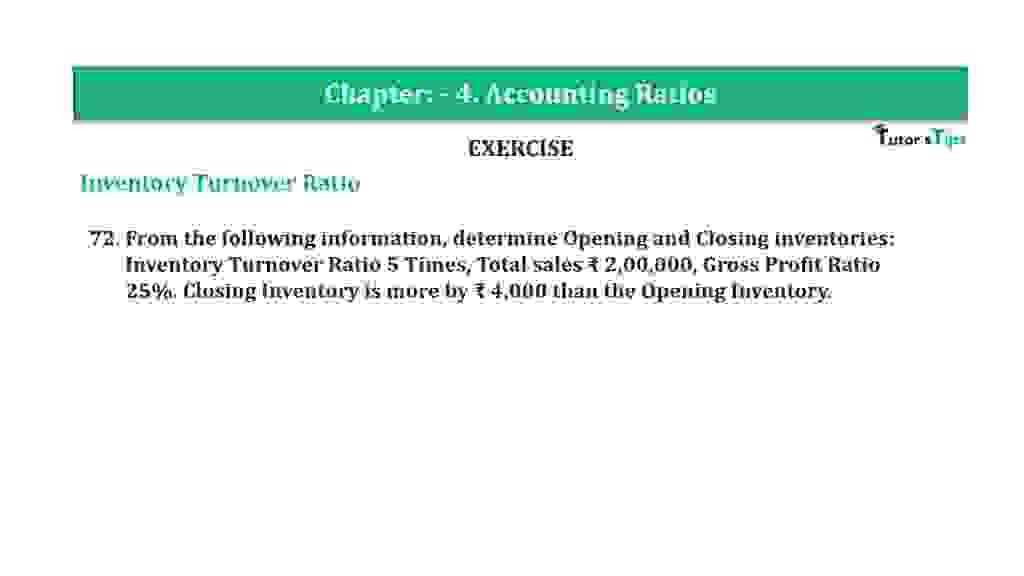# Question 72 Chapter 4 of +2-B – T.S. Grewal 12 ClassQuestion 72 Chapter 4 of +2-B

Interest Coverage Ratio

72. From the following information, determine Opening and Closing inventories:
Inventory Turnover Ratio 5 Times, Total sales ₹ 2,00,000, Gross Profit Ratio 25%. Closing Inventory is more by ₹ 4,000 than the Opening Inventory.

### The solution of Question 72 Chapter 4 of +2-B: –

 Total Sales = Rs. 2,00,000 Gross Profit = 25% on Sales
 Gross Profit = Rs.2,00,000 x 25 100 = Rs. 50,000
 Cost of goods sold = Total Sales – Gross Profit = Rs. 2,00,000 – Rs. 50,000 = Rs. 1,50,000

 Inventory Turnover Ratio = Cost of goods sold Average Inventory

 5 = 1,50,000 Average Inventory
 Average Inventory = Rs. 1,50,000 / 5 = Rs. 30,000 Opening Inventory = x Closing Inventory = X + Rs. 4,000

 Average Inventory = Opening Inventory +Closing Inventory 2
 Rs. 30,000 = X + (X+ Rs. 4,000) 2

 Rs. 60,000 = 2x + Rs. 4,000 2x = Rs. 60,000 – Rs. 4,000 x = Rs. 56,000 / 2 x = Rs. 28,000 Opening Inventory = Rs. 28,000 Closing Inventory = Rs. 28,000 + Rs. 4,000 = Rs. 32,000

Balance Sheet: Meaning, Format & Examples

Thanks, Please Like and share with your friends

Comment if you have any question.

Also, Check out the solved question of previous Chapters: –

### T.S. Grewal’s Double Entry Book Keeping (Vol. II: Accounting for Companies)T.S. Grewal’s Analysis of Financial Statements